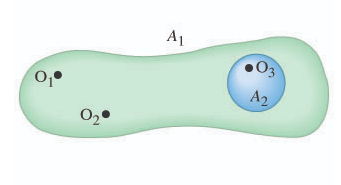# Problem: In (Figure 1) , two objects, O1 and O2, have charges +3.5μC and −1.5μC, respectively, and a third object, O3, is electrically neutral.(a) What is the electric flux through the surface A1 that encloses all three objects?(b) What is the electric flux through the surface A2 that encloses the third object only?

###### FREE Expert Solution

Gauss' law:

$\overline{){{\mathbf{\varphi }}}_{{\mathbf{E}}}{\mathbf{=}}{\mathbf{\oint }}{\mathbf{E}}{\mathbf{·}}{\mathbf{d}}{\mathbf{A}}{\mathbf{=}}\frac{{\mathbf{q}}_{\mathbf{e}\mathbf{n}\mathbf{c}}}{{\mathbf{\epsilon }}_{\mathbf{0}}}}$

(a)

qenclosed = q1 + q2 + q3 = 3.5μC −1.5μC + 0 = +2.0 μC

94% (472 ratings)###### Problem Details

In (Figure 1) , two objects, O1 and O2, have charges +3.5μC and −1.5μC, respectively, and a third object, O3, is electrically neutral.
(a) What is the electric flux through the surface A1 that encloses all three objects?
(b) What is the electric flux through the surface A2 that encloses the third object only?